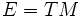# Space of metrics on a bundle

## Definition

Let$M$ be a differential manifold and$E$ be a vector bundle. The space of metrics on$E$ is the set of all possible ways of giving$E$ the structure of a metric bundle.

This can be viewed as a subset of the space of sections of$Sym^2(E^*)$.

In the particular case where$E = TM$, we get the space of Riemannian metrics.

## Facts

### Gauge group acts on the space of metrics

The gauge group of the vector bundle$E$ acts on the space of metrics of$E$.